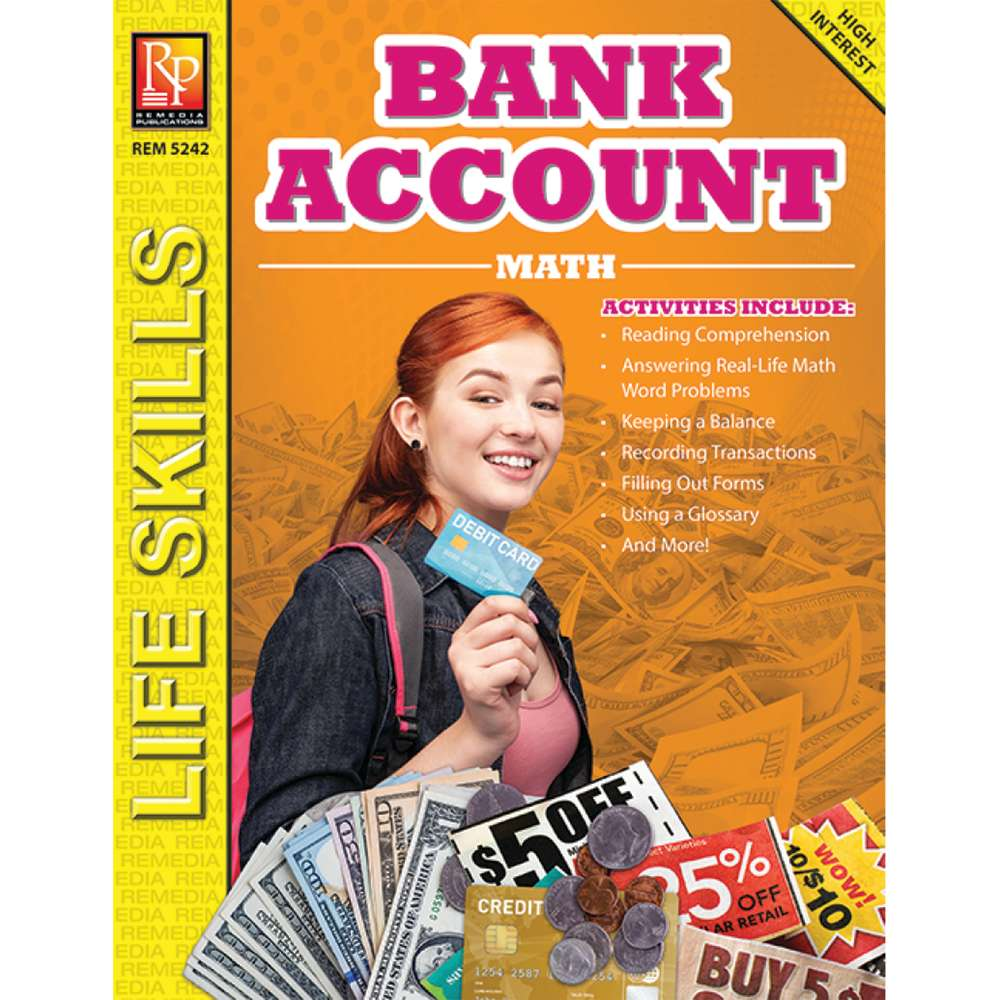•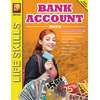•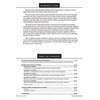•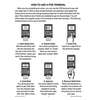•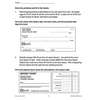•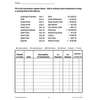Bank Account Math

Product Number: REM5242
\$10.99
A really practical application of math. Each book includes activities such as reading comprehension, filling out forms, using...
Quantity

A really practical application of math. Each book includes activities such as reading comprehension, filling out forms, using a glossary, and answering math word problems. The multi-step word problems cover adding and subtracting money, multiplying and dividing money, figuring percentages, working with large numbers, and more. This life skills program will help students master math skills that are essential to everyday life!

Students practice applying their math skills in addition, subtraction, multiplication, division, percents, fractions, and decimals. They also gain valuable, practical knowledge about the important survival skill of handling money and using a bank account, debit card, withdrawing and depositing money, and more. This 64-page book includes a glossary and an answer key.

REM5242